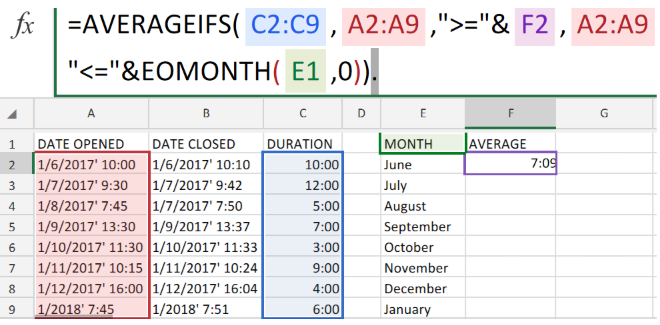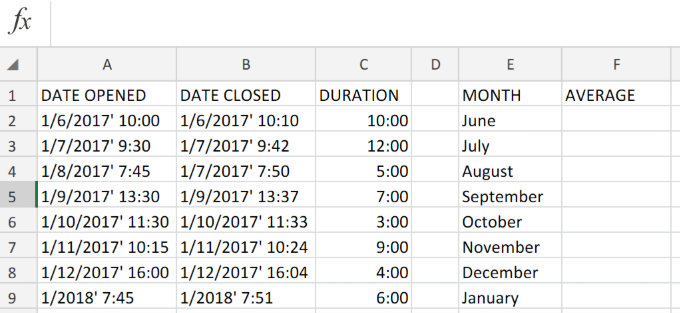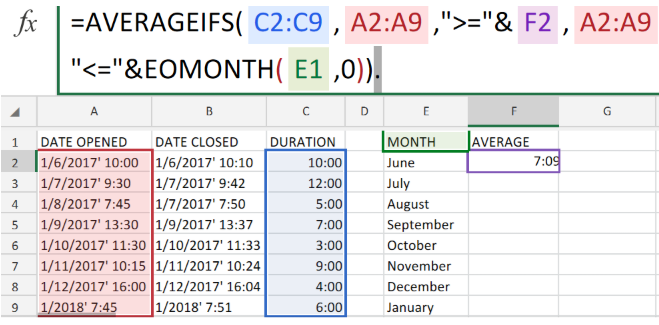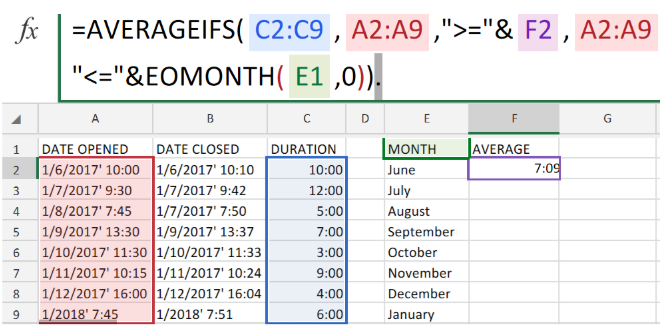Get instant live expert help with Excel or Google Sheets“My Excelchat expert helped me in less than 20 minutes, saving me what would have been 5 hours of work!”

#### Post your problem and you’ll get Expert help in seconds.

Your message must be at least 40 characters
Our professional Expert are available now. Your privacy is guaranteed.

# How to Calculate the Average Response Time Per Month in ExcelFigure 1. of Average Response Time Per Month in Excel.

In order to determine Average Response Time per Month, we are going to use the AVERAGEIFS Function. This tutorial will step through the process of calculating the average response time within a month.

## Generic Formula

`=AVERAGEIFS(durations,dates,">="&A1,dates,"<="&EOMONTH(A1))`

## How to Use the AVERAGEIFS Function in Excel.

The Excel AVERAGEIFS Function operates by using the cell ranges we have specified to determine response times.

We can achieve this by following three simple steps;

1. Collate the data values available in our worksheet. Label the columns appropriately.

In the example illustrated below, we are working with the monthly delivery dates and times for a courier firm.Figure 2. of Delivery Schedule in Excel.

1. Enter the following formula syntax into the formula bar for cell F2;

`=AVERAGEIFS(C2:C9,A2:A9,">="&F2,A2:A9,"<="&EOMONTH(E1,0))`Figure 3. of Average Response Time per Month in Excel.

Excel will reference the delivery dates and times then determine the average time of response, to be displayed in column F of our worksheet.

1. Modify and Copy the formula syntax in cell F2 above, into the other cells in column F to get the desired results.

## Note

• In the example illustrated above, our dates cell Range is A2:A9 (monthly).
• In the example illustrated above, our duration cell range is C2:C9 (minutes).

In our example, we have configured the AVERAGEIFS operation syntax to average monthly durations by using two specific criteria

(1) matching any dates that are greater than or equal to the first day of the month,

(2) matching any dates that are less than or equal to the last day of the month.Figure 4. of Final Result.

## Instant Connection to an Expert through our Excelchat Service:

Our live Excelchat Service is here for you. We have Excel Experts available 24/7 to answer any Excel questions you may have. Guaranteed connection within 30 seconds and a customized solution for you within 20 minutes.

Are you still looking for help with the Average function? View our comprehensive round-up of Average function tutorials here.

### Did this post not answer your question? Get a solution from connecting with the expert.Another blog reader asked this question today on Excelchat:
Solution examplesI just need to edit this formula, {=IFERROR(AVERAGEIFS(B6:D6,F6:H6,"FALSE"),AVERAGEIF(B6:D6,"<>0"))}, to return "-" or "0" if there is nothing in column D. I can share the entire worksheet if need be.
Solved by V. A. in 47 mins## Subscribe to Excelchat.coAnother blog reader asked this question today on Excelchat: